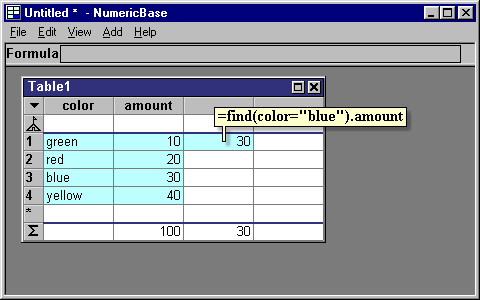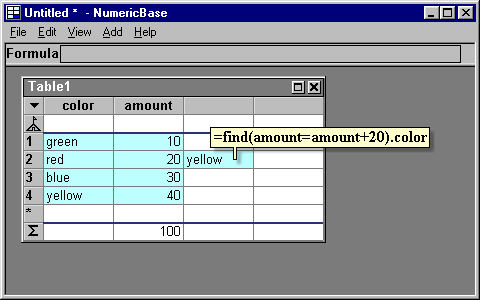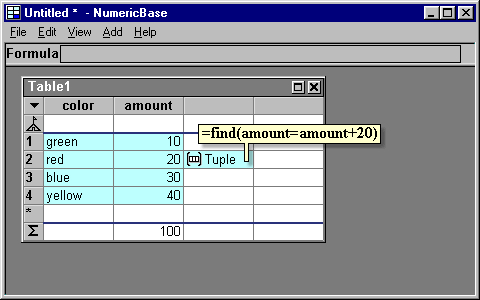# The "find" function

The "find" function
This function scans all the different rows of a table and returns a reference to the first row that matches a criteria. The criteria is a formula passed to this function as the only argument. The function evaluates the criteria formula in the context of the different rows, and return reference to the first row where the criteria evaluates to the "true" logical value (after conversion, if necessary)

For example, the formula =find(color="blue").amount of the following screenshot, scans all the rows of the table, and evaluates the criteria color="blue" at the context of the different rows. The first row that matches the criteria is row number 3. Therefore, the value of the entire formula is 30 - the value of the amount column from that row(row number 3).Screenshot: the find function.

Different contexts
If the criteria formula has comparison operators (it does not have to), then the find function evaluates the different parts of the formula at different contexts. The formula part that is left of the comparison operator is evaluated at the context of the different rows, and the formula part that is right to a comparison operator is evaluated at the context of the current row. This let you combine values from the current row and match them against values from the different rows of the table.

For example, the formula =find (amount=amount+20).color of the following screenshot, first evaluates the formula part amount+20 at the context of the current row (is equals 40), and then matches this criteria, using the equal operator, against the different rows of the table. The first row that matches this criteria is row number 4. The formula then extract the color value from the resulting row reference, so the final result is yellow.Screenshot: The find function evaluates different parts of the criteria in different contexts

If the formula does not extract information from the find function result, then the result of the formula is a reference to the found row:Screenshot: the find function returns a row reference